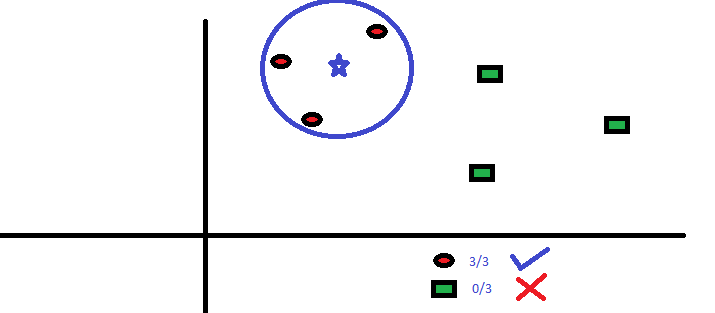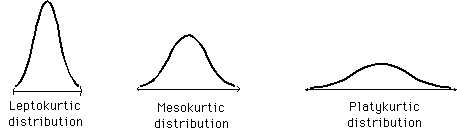# Some common Machine Learning, Statistics and Data Science terms starts with K

## K

[su_table]

### Word ### Description
K-Means It is a type of unsupervised algorithm which solves the clustering problem. It is a procedure which follows a simple and easy way to classify a given data set through a certain number of clusters (assume k clusters). Data points inside a cluster are homogeneous and heterogeneous to peer groups.|
|Keras|Keras is a simple, high-level neural network library, written in Python. It is capable of running on top of Tensorflow and Theano. This is done to make design and experiments with Neural Networks easier.

Following are some important features of Keras:

• User friendliness
• Modularity
• Easy extensibility
• Work with Python|
|kNN|K nearest neighbors is a simple algorithm that stores all available cases and classifies new cases by a majority vote of its k neighbors. The case being assigned to the class is most common amongst its K nearest neighbors measured by a distance function.

These distance functions can be Euclidean, Manhattan, Minkowski and Hamming distance. First three functions are used for continuous function and fourth one (Hamming) for categorical variables. If K = 1, then the case is simply assigned to the class of its nearest neighbor. At times, choosing the value for K can be a challenge while performing KNN modeling.|